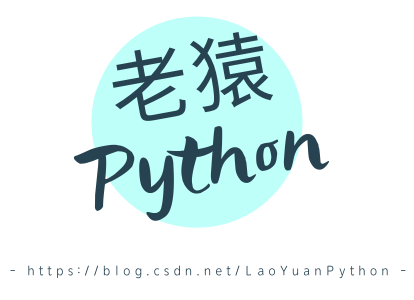# Python3 print 变量打印输出功能后面隐含的几个知识点#### 知识点一：Python3 所有类型都是类

>>> i=1>>> i.__dir__()['__repr__', '__hash__', '__getattribute__', '__lt__', '__le__', '__eq__', '__ne__', '__gt__', '__ge__', '__add__', '__radd__', '__sub__', '__rsub__', '__mul__', '__rmul__', '__mod__', '__rmod__', '__divmod__',  '__rdivmod__', '__pow__', '__rpow__', '__neg__', '__pos__', '__abs__', '__bool__', '__invert__',  '__lshift__', '__rlshift__', '__rshift__', '__rrshift__', '__and__', '__rand__', '__xor__', '__rxor__',  '__or__', '__ror__', '__int__', '__float__', '__floordiv__', '__rfloordiv__', '__truediv__',  '__rtruediv__', '__index__', '__new__', 'conjugate', 'bit_length', 'to_bytes', 'from_bytes',  'as_integer_ratio', '__trunc__', '__floor__', '__ceil__', '__round__', '__getnewargs__', '__format__',  '__sizeof__', 'real', 'imag', 'numerator', 'denominator', '__doc__', '__str__', '__setattr__',  '__delattr__', '__init__', '__reduce_ex__', '__reduce__', '__subclasshook__', '__init_subclass__',  '__dir__', '__class__']>>> i.__class__<class 'int'>>>> i.__str__()'1'>>> i.__repr__()'1'>>> 

#### 知识点二：Python3 中所有自定义类都是 object 类的子类

>>> class person:  def __init__(self,id,name):    self.id,self.name=id,name    >>> marry = person(12345678,'marry')>>> print(marry)<__main__.person object at 0x0000000002C765B0>>>> str(marry)'<__main__.person object at 0x0000000002C765B0>'>>> marry.__dir__()['id', 'name', '__module__', '__init__', '__dict__', '__weakref__', '__doc__', '__repr__', '__hash__', '__str__', '__getattribute__', '__setattr__', '__delattr__', '__lt__', '__le__', '__eq__', '__ne__',  '__gt__', '__ge__', '__new__', '__reduce_ex__', '__reduce__', '__subclasshook__', '__init_subclass__',  '__format__', '__sizeof__', '__dir__', '__class__']>>> marry.__dict__{'id': 12345678, 'name': 'marry'}>>> marry.__class__<class '__main__.person'>>>> marry.__class__.__base__<class 'object'>>>> person.__base__<class 'object'>>>> 

#### 知识点三：所有类型及类都有__str__和__repr__实例方法

Python 中__str__方法是为了给最终用户提供实例对象信息，__repr__方法是给开发人员提供实例对象信息。__str__应该提供用户容易理解的信息，将属性代码翻译成客户理解的文字说明，__repr__应该提供开发者容易使用的信息，最好是能够直接使用输出的信息直接拷贝部分或全部就能重定义一个对象，这些信息类似于集成环境下 debug 看内存数据对象的格式差不多。

#### 知识点五：在 print(变量)时输出的是实例方法__str__函数返回的内容

>>> class person:  def __init__(self,id,name):    self.id,self.name=id,name  def __str__(self):    return self.name>>> jk = person(23455,'Jack')>>> str(jk)'Jack'>>> repr(jk)'<__main__.person object at 0x0000000002C76880>'>>> print(jk)Jack

#### 知识点六：自定义类对__str__与__repr__方法的处理

1. 如果自定义类自己重写了__str____repr__方法，则会按重写的方法执行；

2. 如果自定义类没有定义__str__方法但定义了__repr__方法，调用__str__方法实际上会调用__repr__方法输出；

3. 如果自定义类没有定义__repr__方法但定义了__str__方法，调用__str__方法会按重写的方法执行，调用__repr__方法实际上会调用 obejct 类的__repr__方法；

4. 如果自定义类没有重写了__str____repr__方法，则会按 object 类的__str____repr__方法执行。

#### 小结：### 老猿Python

CSDN 2020年博客之星季军、高级程序员、超50万行C语言项目开发经验 擅长领域：Python语言、PyQt界面程序开发、Moviepy音视频剪辑、OpenCV-Python图像处理、爬虫、5G、区块链、人工智能数学基础

## 评论